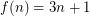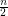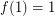# integer sequence

## The 3n+1 conjecture ★★★

Author(s): Collatz

Conjecture   Letifis odd andifis even. Let. Assume we start with some numberand repeatedly take theof the current number. Prove that no matter what the initial number is we eventually reach.

Keywords: integer sequence